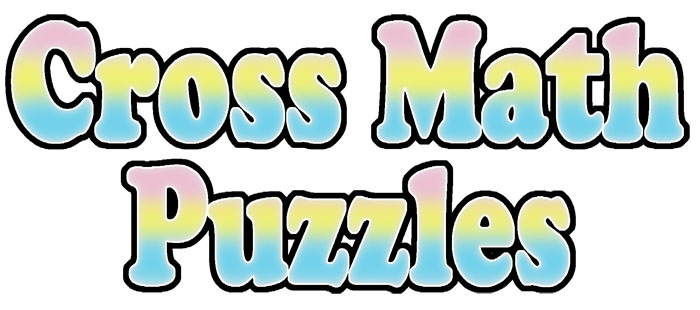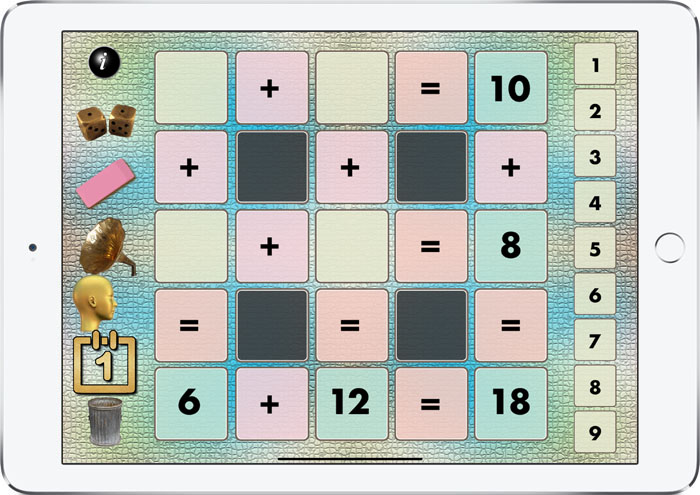Use Cross Math Puzzles to Provide Math Practice in a Fun Way...An Interactive App to Inspire Kids to Enjoy Math and Problem Solving...Fun and challenging! Practice your math skills and logical thinking abilities with Cross Math Puzzles. Cross Math Puzzles involve using whole numbers and integers to solve addition, subtraction and multiplication problems. The object of Cross Math Puzzles is to place the correct numbers in the empty cells of the puzzle so that the horizontal and vertical equations are true. Puzzles are randomly generated so an unlimited supply of puzzles are available. Cross Math Puzzles are a great for elementary students to practice their math skills. The app provides five different types of problems. The first level uses sums for whole numbers from 1 to 9. At level two a mix of addition and subtraction problems are presented. Level three involves multiplication of numbers from 1 to 9. When level four is selected, addition problems for numbers between -4 and +4. At level five addition and subtraction problems for integers between -4 and +4 are presented. Speech and sound effects make using Cross Math Puzzles fun for kids. A scoreboard keeps track of how many puzzles the student has solved. The app is a available at a discount for volume purchasing on Apple School Manager.   [ See also Geometry Portal AR, Algebra Portal AR and Geometry AR ] \$1.99!Special features make using the Cross Math Puzzles app fun: 1. Students are motivated to solve the puzzle and do so by practicing math skills. 2. Scores are saved in order to show a teacher, parents and friends your progress. 3. Speech and sound effects. 4. Available for iPad iOS and tvOS. 5. Available at a discount to schools participating in Apple's Volume Purchase Program.\$1.99 Now Available on the Apple App Store.